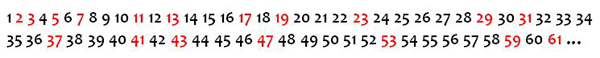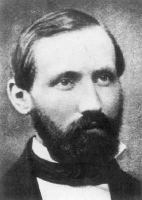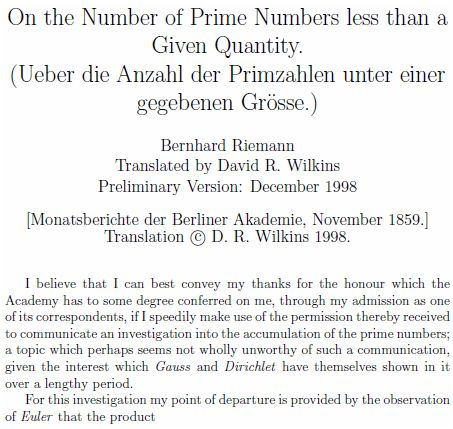No. 2575:
Prime Numbers
Audio

Today, some numbers for the ages. The University of Houston's College of Engineering presents this series about the machines that make our civilization run, and the people whose ingenuity created them.

You can't add apples and oranges, or, so we're told in grade school. It's a way of reminding us we can't add or subtract fractions unless they have a common denominator. And when we learn how to find a common denominator, we bump into some very famous numbers — the primes.

A number is prime if it can be divided by exactly two distinct numbers — itself and one. Seven is prime. Divide it by any whole number other than one or seven and there's a fraction left over. Six is not prime. It can be divided not just by one and six, but by two and three.

We got interested in primes long ago. Euclid's Elements contains some simple yet ingenious proofs about prime numbers — proofs that are important for working with fractions. TheSieve of Eratosthenes, discovered more than 2200 years ago, is an algorithm for finding prime numbers. It's one of the earliest known algorithms of any kind.But it wasn't until the seventeenth century that mathematicians began a deep investigation of prime numbers. Much of the investigation went along these lines. If we make a list of the counting numbers — 1, 2, 3 and so on — do the prime numbers show patterns?It's an intriguing question, and one that captured the imagination of a long list of renowned mathematicians. Fermat. Euler. Gauss. These are just a few of the names. And the more that people studied prime numbers, the more fashionable it became. In 1859, Bernhard Riemann published a now famous mathematical paper where he made an amazing conjecture about patterns in prime numbers. What is now called the Riemann Hypothesis is one of the seven celebrated millennium problems put forth by the Clay Mathematics Institute. Proving or disproving it carries a million dollar prize.

The study of prime numbers was long considered an exercise in pure mathematics — mathematics that's measured by aesthetics, not usefulness. But that abruptly changed in 1978 when three researchers described a special way to scramble and unscramble coded messages with the help of prime numbers. Their method's perfect for the Internet, and put prime numbers at the very heart of electronic commerce. Thanks to prime numbers, we can safely send information like our credit card number without fear that someone will steal it.Prime numbers provide a wonderful thread through history. From ancient Greece through generations of mathematicians, prime numbers still challenge us with fascinating mathematical questions. And we all get a chance to meet these beguiling building blocks when we learn about apples and oranges.

I'm Andy Boyd at the University of Houston, where we're interested in the way inventive minds work.

(Theme music)

Notes and references:

For a related episode, see 1994, THEORY AND ENCRYPTION

At times in history, 1 has been defined as prime. At other times, it hasn't. As it's a matter of definition, there's no right or wrong. Today, 1 is commonly viewed in a class by itself: prime numbers are numbers divisible by exactly two distinct numbers (1 and itself, which can't be 1), composite numbers are all other numbers except 1, and 1 stands alone.

By putting 1 in a separate category, it simplifies the statement of many results. For example, the Fundamental Theorem of Arithmetic known to the early Greeks can be stated "all numbers can be written as a unique product of primes." We have 60 = 2 x 2 x 3 x 5, and no other combination of prime numbers yields the product 60. If 1 was prime, then the Fundamental Theorem wouldn't hold as stated, since 60 = 2 x 2 x 3 x 5 and 60 = 1 x 2 x 2 x 3 x 5. If 1 is treated as prime, then the Fundamental Theorem would need to be stated in a fashion such as "excluding the factor 1, all numbers can be written as a unique product of primes."

The Prime Pages. A wonderful compilation of all things prime from the University of Tennessee, Martin, Web site: http://primes.utm.edu. Accessed January 25, 2010.

R. Rivest, A. Shamir, and L. Adleman. "A Method for Obtaining Signatures and Public-Key Cryptosystems." Communications of the ACM, Vol. 20, No. 2, pp. 120-126.

The picture of Bernhard Riemann is from Wikimedia Commons.